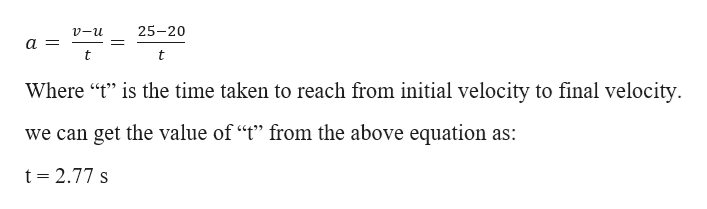# A car is moving at a velocity of 20 m/s. The car then accelerates uniformly at 1.8 m/s2. The car continues at the same acceleration until it reaches a velocity of 25 m/s, which is the legal speed limit. What is the distance the car travels in while accelerating?

Question
3 views

A car is moving at a velocity of 20 m/s. The car then accelerates uniformly at 1.8 m/s2. The car continues at the same acceleration until it reaches a velocity of 25 m/s, which is the legal speed limit. What is the distance the car travels in while accelerating?

check_circle

Step 1

Given information:

Initial velocity of the car (u) = 20 m/s.

Final velocity of the car (v) = 25 m/s.

Acceleration of the car (a) = 1.8 m/s2

Step 2

From the definition of average acceleration (a), we can relate these quantities as:help_outlineImage Transcriptionclose25-20 v-u 1 t t Where "t is the time taken to reach from initial velocity to final velocity we can get the value of "t" from the above equation as: t 2.77 s fullscreen
Step 3

From the 2nd equation of motion we find the distance (s) travelled by t...

### Want to see the full answer?

See Solution

#### Want to see this answer and more?

Solutions are written by subject experts who are available 24/7. Questions are typically answered within 1 hour.*

See Solution
*Response times may vary by subject and question.
Tagged in

### Kinematics